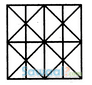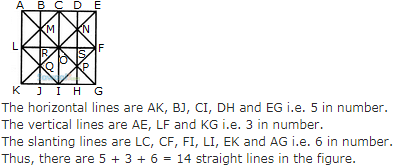# Non Verbal Reasoning (Odd One Out) MCQ - 3

## 10 Questions MCQ Test Reasoning Aptitude for Competitive Examinations | Non Verbal Reasoning (Odd One Out) MCQ - 3

Description
Attempt Non Verbal Reasoning (Odd One Out) MCQ - 3 | 10 questions in 10 minutes | Mock test for LR preparation | Free important questions MCQ to study Reasoning Aptitude for Competitive Examinations for LR Exam | Download free PDF with solutions
QUESTION: 1

### Find the minimum number of straight lines required to make the given figure.Solution:QUESTION: 2

Solution:
QUESTION: 3

### Find the odd one out

Solution:
QUESTION: 4

Find the odd one out

Solution:
QUESTION: 5

Find the odd one out

Solution:
QUESTION: 6

Find the odd one out

Solution:
QUESTION: 7

Find the odd one out

Solution:
QUESTION: 8

Find the odd one out

Solution:
QUESTION: 9

Find the odd one out

Solution:
QUESTION: 10

Find the odd one out

Solution:Use Code STAYHOME200 and get INR 200 additional OFF Use Coupon Code